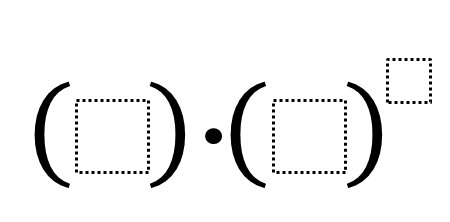Home > Grade 8 > Expressions & Equations > Exponents and Order of Operations

# Exponents and Order of Operations

Directions: Find 3 positive integers that add up to 10. Place each number into one of the blanks to find the largest possible result.### Hint

Which part of the expression should be simplified first?
Which of the three boxes should get the largest number?

1 * 4^5

### Extensions

There are many extensions with the exact same prompt:
1. What’s the smallest possible result?
2. What if you’re working with all integers greater than (-5)?
3. How close can you get to 0 without hitting it exactly?

Source: Zack Miller (@zmill415)

## Square Root Expression

Directions: Use the digits 1 to 9, at most one time each, to fill in …

1.(2×3)^5 results in a larger number than (1×4)^5

2.Oops, didn’t see the comment thread above. The order of operations says we do the exponents first, so:
2 x 3^5 < 1 * 4^5

3.is it right or wrong so which one is bigger?

4.I don’t get it there is no number to work with it , weirdo is out